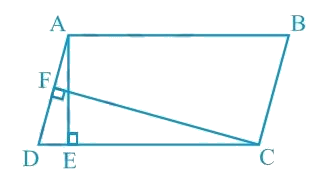In the verge of coronavirus pandemic, we are providing FREE access to our entire Online Curriculum to ensure Learning Doesn't STOP!

# Ex.9.2 Q1 Areas of Parallelograms and Triangles Solution - NCERT Maths Class 9

Go back to  'Ex.9.2'

## Question

In the given figure, $$ABCD$$ is parallelogram, $$AE ⊥ DC$$ and $$CF ⊥ AD$$ . If $$AB = 16\; \rm{cm}$$, $$AE = 8 \;\rm{cm}$$ and $$CF = 10\;\rm{cm}$$, find $$AD$$.Video Solution
Areas Of Parallelograms And Triangles
Ex 9.2 | Question 1

## Text Solution

What is known?

ABCD is parallelogram $${AE}\bot {DC}$$ , and  $${CF}\bot {AD}$$ . If $$AB = 16 \,\rm{cm}, AE = 8 \,\rm{cm}$$ and $$CF = 10\, \rm{cm}$$, find $$AD$$.

What is unknown?

Length of $$AD$$.

Reasoning:

Area of a parallelogram $$ABCD$$

\begin{align}&= CD \!\!\times\!\!{ AE}\,\\&=\,\,{AD}\,\!\!\times\!\!\,{CF}\end{align}

Steps:

Given:

$$AB = 16\; \rm{cm}$$.

$$AE = 8 \;\rm{cm}$$.

$$CF = 10\;\rm{cm}$$.

In parallelogram $$ABCD$$, $$CD = AB = 16 \;\rm{cm}$$

[Opposite sides of a parallelogram are equal]

We know that,

Area of a parallelogram $$=$$ Base $$×$$ Corresponding altitude

Therefore, Area of a parallelogram \begin{align} {\rm{ABCD = CD \times AE}}\,{\rm{ = }}\,\,{\rm{AD}}\,\,{\rm{ \times }}\,\,{\rm{CF}}\end{align}

Here $$CD$$ and $$AD$$ act as base and $$AE ⊥ CD$$ , $$CF ⊥ AD$$ are the corresponding altitudes.

Therefore,\begin{align} {AD} &= \frac{{{{CD }} \times {{ AE}}}}{{{{CF}}}}\end{align}

Substituting the values for $$CD$$ , $$AE$$ and $$CF$$, we get

\begin{align}{AD}&=\frac{16 \times 8}{10} {cm}=12.8 {cm}\end{align}

Thus, the length of $$AD$$ is $$12.8 \;\rm{cm}$$.

Video Solution
Areas Of Parallelograms And Triangles
Ex 9.2 | Question 1

Learn from the best math teachers and top your exams

• Live one on one classroom and doubt clearing
• Practice worksheets in and after class for conceptual clarity
• Personalized curriculum to keep up with school